Horin Geophysical Software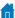Current Position: Home > Horin Geophysical Software > 3D Forward & Inversion

##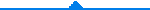SoftwareFeature

Euler 3D Inversion Computation

Horin software provides you with a set of professional inversion tool "Euler 3D inversion computation", which is used to compute field source solutions. This is a potential field inversion method for position estimation of field source in the instance of lacking geology information. By solving Euler homogeneous equation, we can quickly compute the position, depth and boundary of gravity and magnetic field source. It is especially suitable for rapid three-dimensional geology interpretation of potential field. Euler 3D inversion computation function is a practical and reliable interpretation tool for gravity and magnetic data.

Euler homogeneous equation: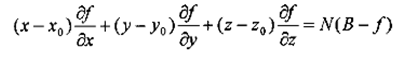Tectonic index (N)Euler solution set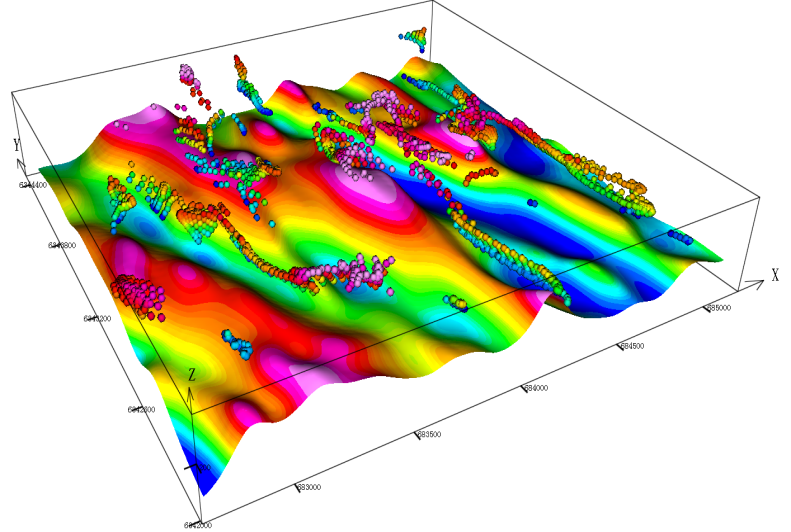map view                                                                                                                       3D view

Euler 3D Inversion Computation

Professional inversion tool "Euler 3D inversion computation", which makes the engineer's geophysical interpretation work become more concise and efficient.

Convenient operation

Euler function in Horin software is an independent module, which is very convenient to operate. The entire process of calculating the Euler result only needs three steps: " finding derivative ", "solving Euler equation" and "filter the solutions". You can obtain Euler 3D inversion compute results in a few minutes. Finally, the map view and 3D view can be quickly displayed using Horin platform function.

Fast calculation

We optimized the algorithm of Euler inversion in Horin software. Euler function can process tens of thousands of grid points in a few minutes.

Single sphere model

We use single sphere model to verify the rationality of Euler algorithm.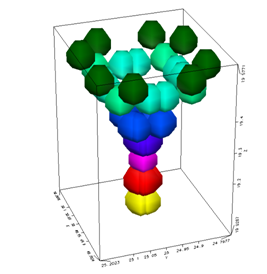Map view of Euler solution set                                                                                                            3D viewX direction derivative                                              Y direction derivative                                                   Z direction derivative

Case display

Case shows the 3D inversion results, you can improve the credibility of Euler solution set by filtering.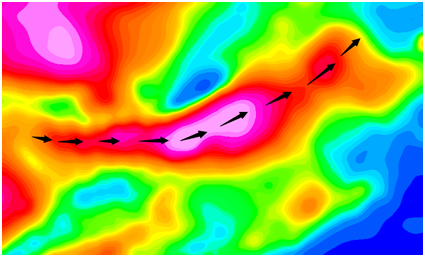Raw sample data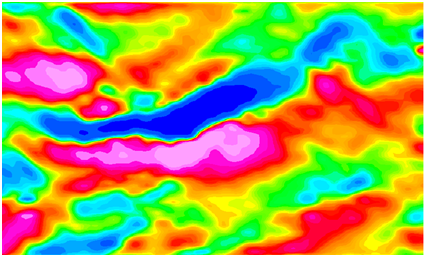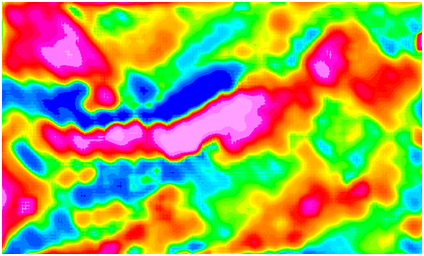X direction derivative                                                Y direction derivative                                                        Z direction derivative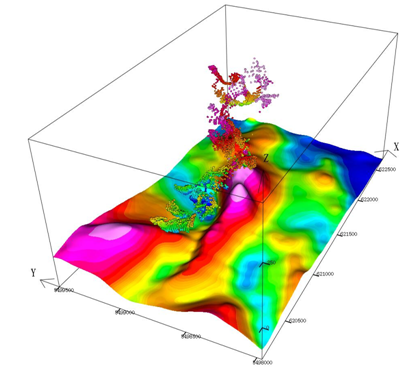Contrast effect of filtering                                                                                                                     3D view